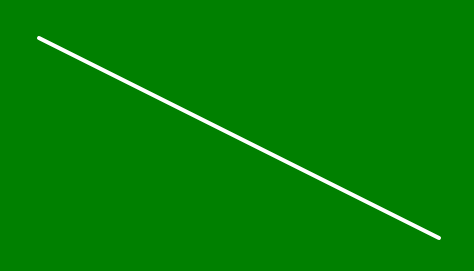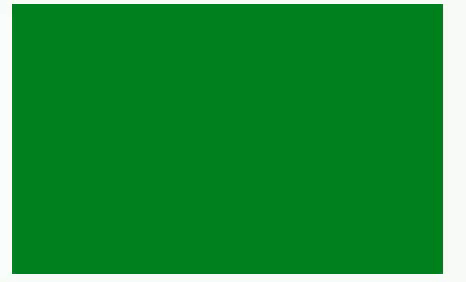GeeksforGeeks App
Open AppBrowser
Continue

## Related Articles

The noLoop() function is used to stop the program after executing the draw() function. The loop() function runs the draw() function again and again. If noLoop() function is used in setup() function then it should be the last line inside the block. If noLoop() function is used then it is not possible to change or access the screen inside event handling functions such as mousePressed() or keyPressed().

Syntax:

`noLoop()`

Below examples illustrate the noLoop() function in p5.js:

Example 1:

 `function` `setup() {``   ` `  ``// Create canvas of given size``  ``createCanvas(500, 300);``   ` `  ``// Set the background color``  ``background(``'green'``);``   ` `  ``// Use noLoop() function``  ``noLoop();``}`` ` `function` `draw() {``   ` `  ``// Set the stroke color``  ``stroke(``'white'``);``   ` `  ``// Set the stroke width``  ``strokeWeight(4);``   ` `  ``// Function to draw the line``  ``line(50, 50, 450, 250);``   ` `}`

Output:Example 2:

 `let l = 0;`` ` `function` `setup() {``   ` `  ``// Create canvas of given size``  ``createCanvas(500, 300);``   ` `  ``// Set the background color``  ``background(``'green'``);`` ` `}`` ` `function` `draw() {``   ` `  ``// Set the stroke color``  ``stroke(``'white'``);``   ` `  ``l = l + 0.5;``  ``if` `(l > width) {``    ``l = 0;``  ``}``   ` `  ``// Function to draw the line``  ``line(l, 0, l, height);``   ` `}`` ` `function` `mousePressed() {``  ``noLoop();``}`` ` `function` `mouseReleased() {``  ``loop();``}`

Output:My Personal Notes arrow_drop_up
Related Tutorials